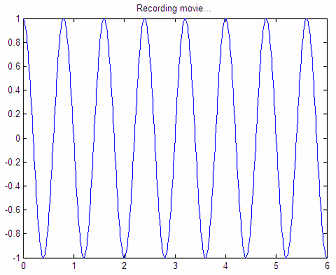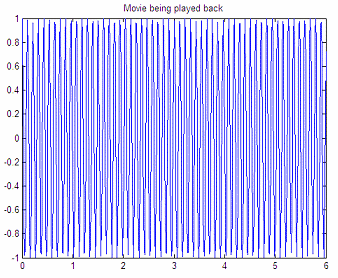Simple Animation in Matlab

 If we have some data representing a system or a function at several time intervals, we may want to take advantage of Matlab’s simple animation capabilities.  We're going to expose the basic method or algorithm for animations. In this example we’re going to work with just three special instructions.

The idea is to store every figure as a frame of the ‘movie’, with each frame stored as a column vector of a matrix, an then play all the frames on the screen.

moviein(nr_frames): we initialize the matrix that will keep the frames, with the number of frames to be generated.

getframe: with this instruction we keep the information of a given figure and ‘load’ a temporary matrix with information.

movie(matrix, times, FPS): this is used to play the movie after its generation. Parameter ‘matrix’ is the saved data, ‘times’ the number of times that the movie will be played back, and ‘FPS’ means ‘frames per second’ (the default is 12).

Let’s try this simple animation example:

% Define number of frames
nr_fr = 10;
% Initialize matrix using 'moviein'
frames = moviein(nr_fr);

% Generate frames with any plotting function.
% We use a cosine with variable frequency.

t = 0 : .01 : 6;
f = 1;

for i = 1 : nr_fr
f = f * 1.25; w = 2 * pi * f;
y = cos(w*t);
plot(t, y);
title(
'Recording movie...')

% Get every frame with 'getframe' and load the appropriate       % matrix.
frames(:, i) = getframe;
end

% Save the matrix so that this movie can be loaded later
save frames

This is the first frame of the recording:Now you can play back the movie:

% Play the movie once, 2 FPS.
title('Movie being played back...')
movie(frames, 1, 2)

This is the last frame of the animation:Done!

From 'Simple Animation' to home

From 'Simple Animation' to 2D Menu

 Top Animation: comets 3D Animation